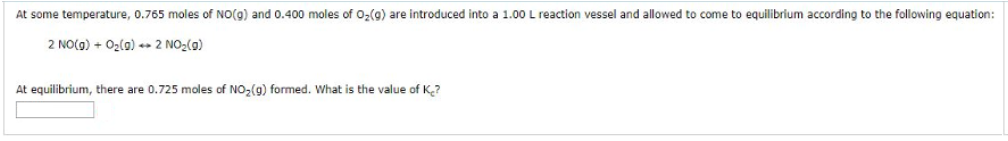# Problem: At some temperature, 0.765 moles of NO(g) and 0.400 moles of O2(g) are introduced into a 1.00 L reaction vessel and allowed to come to equilibrium according to the following equation:2NO(g) + O2(g) → 2 NO2(g) At equilibrium, there are 0.725 moles of NO2(g) formed. What is the value of Kc?

###### FREE Expert Solution
100% (91 ratings)###### Problem Details

At some temperature, 0.765 moles of NO(g) and 0.400 moles of O2(g) are introduced into a 1.00 L reaction vessel and allowed to come to equilibrium according to the following equation:

2NO(g) + O2(g) → 2 NO2(g)

At equilibrium, there are 0.725 moles of NO2(g) formed. What is the value of Kc